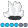Eva
Quartz | Level 8

## 100% per bar in a subgrouped and grouped vbar possible?

Dear all,

I have a proc gchart with vbar, group and subgroup. The type is percent. If I don't add anything then 100% is the whole chart. If I add the option g100 then 100% is within one group. Now, my customer wants 100% per bar in this chart. Is that possible?

Best wishes

Eva

1 ACCEPTED SOLUTION

Accepted Solutionsdata_null__

## Re: 100% per bar in a subgrouped and grouped vbar possible?

Is that it?

proc summary data=sashelp.cars (where=(make in ("Audi","BMW"))) nway;

class make type cylinders;
var enginesize;
output out=summary sum=;
run;
proc freq data=summary noprint;

by make type;
tables cylinders / out=pct nocum;

weight enginesize;
run;
proc gchart data=pct;
vbar make / group = type

subgroup = cylinders

sumvar=percent

type=sum
;
run;

quit;
5 REPLIES 5

## Re: 100% per bar in a subgrouped and grouped vbar possible?

A code example of what you attempted would be helpful.Eva
Quartz | Level 8

## Re: 100% per bar in a subgrouped and grouped vbar possible?

proc gchart data=sashelp.cars (where=(make in ("Audi","BMW")));

vbar make / freq = enginesize

group = type

subgroup = cylinders

type=percent

g100 /* effect: one group=100% , but I need one bar=100% */

;

run;

quit;data_null__

## Re: 100% per bar in a subgrouped and grouped vbar possible?

Is that it?

proc summary data=sashelp.cars (where=(make in ("Audi","BMW"))) nway;

class make type cylinders;
var enginesize;
output out=summary sum=;
run;
proc freq data=summary noprint;

by make type;
tables cylinders / out=pct nocum;

weight enginesize;
run;
proc gchart data=pct;
vbar make / group = type

subgroup = cylinders

sumvar=percent

type=sum
;
run;

quit;Eva
Quartz | Level 8

## Re: 100% per bar in a subgrouped and grouped vbar possible?

Yes! I always searched for a vbar Option... But a proc freq before the proc gchart is best 🙂

## Re: 100% per bar in a subgrouped and grouped vbar possible?

I'm not sure quite what you want but if each bar should total to 100 across the cylinder count you may need to process your data a bit before taking it to GCHART.

Maybe this is what you are looking for?

proc freq data=sashelp.cars (where=(make in ("Audi","BMW"))) noprint;
table make* type *cylinders/ outpct out=work.cars;
run;

proc gchart data=work.cars (where=(make in ("Audi","BMW")));
vbar make /    sumvar=Pct_row
group = type
subgroup = cylinders
type=sum
;
label pct_row='Percent of Engine Cylinders' ;
run;
quit;

Discussion stats
• 5 replies
• 635 views
• 0 likes
• 3 in conversation